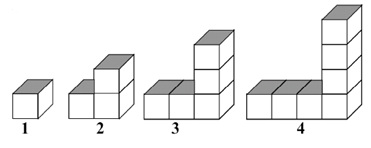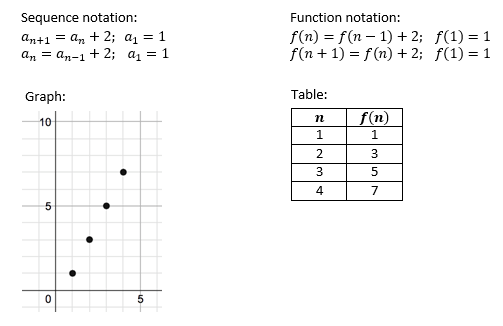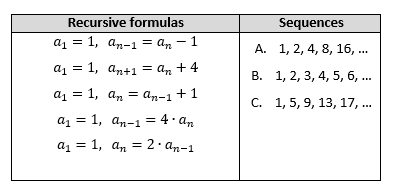# Exponents and Exponential Functions

## Objective

Describe and analyze sequences given their recursive formulas.

## Common Core Standards

### Core Standards

?

• F.BF.A.2 — Write arithmetic and geometric sequences both recursively and with an explicit formula, use them to model situations, and translate between the two forms. Modeling is best interpreted not as a collection of isolated topics but in relation to other standards. Making mathematical models is a Standard for Mathematical Practice, and specific modeling standards appear throughout the high school standards indicated by a star symbol (★). The star symbol sometimes appears on the heading for a group of standards; in that case, it should be understood to apply to all standards in that group.

• F.IF.A.2 — Use function notation, evaluate functions for inputs in their domains, and interpret statements that use function notation in terms of a context.

• F.IF.A.3 — Recognize that sequences are functions, sometimes defined recursively, whose domain is a subset of the integers. For example, the Fibonacci sequence is defined recursively by f(0) = f(1) = 1, f(n+1) = f(n) + f(n-1) for n ≥ 1.

?

• 8.F.B.4

## Criteria for Success

?

1. Define sequences as a set of elements ordered in a way that they are labeled with consecutive positive integers starting with $1$
2. Understand sequences as functions.
3. Describe features of sequences given their recursive formula, written in sequence notation and in function notation.
4. Evaluate sequences using their recursive formula.
5. Use precise language to describe terms in sequences, in both sequence notation and in function notation.
6. Understand there are two ways to write a formula for a sequence: recursively and explicitly.

## Tips for Teachers

?

Sequence notation is new for students and can be challenging when they are also trying to understand recursive formulas. Encourage students to describe and explain sequences out loud with their peers using language such as “previous term” and “next term.”

## Anchor Problems

?

### Problem 1

A pattern of cubes is shown below.a.   Describe the pattern you see.

b.   Write the pattern as a numerical sequence.

c.   What is the value of term ${a_6}$?

d.   What is the value of the term ${f(8)}$?

e.   If you knew the value of the ${n^{th}}$ term, describe how you would find the ${(n+1)^{th}}$ term and the ${(n-1)^{th}}$ term.

#### References

Visual Patterns Visual: Pattern #2

Pattern #2 is made available by Jed Butler on Visual Patterns. ©2017 visualpatterns.org. Accessed May 22, 2018, 9:55 a.m..

### Problem 2

Below are several ways to represent the sequence shown in Anchor Problem #1. Describe how each representation models the sequence.### Problem 3

Which sequence is described by each recursive formula? Some formulas may describe the same sequence, and some formulas may not describe any of the sequences.## Problem Set

?

The following resources include problems and activities aligned to the objective of the lesson that can be used to create your own problem set.

• Include problems where students are given sequences in recursive formula form, and have students write the sequence, describe, graph, create a table, etc.

${f(1)=2}$ ,${{f(n)}=f(n-1)+3}$
3. Explain how to find the term ${f(n+1)}$ if you are given the value of the term ${f(n)}$.